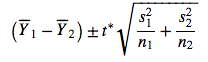# R Instructions: Two Independent Samples

Recall that we were testing

H0: μ1 - μ2 = 0

Ha: μ1 - μ2 > 0

and we found using R that the test statistic was 5.31 with a P-value of 0.000. Based on the small P-value, we rejected Ho and concluded that males 20-29 years old weigh more, on average, than males 75+ years old. It would be interesting to follow up this conclusion and estimate how much more males 20-29 years old weigh, on average. The 95% confidence interval for μ1 - μ2 does exactly that, and is given by the formulaAs a reminder, here again is the summary of the study results: